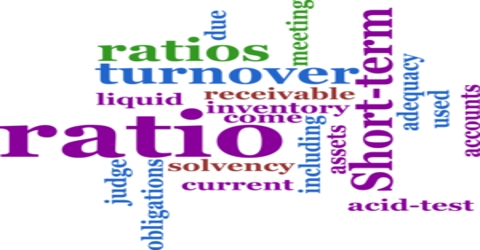# Significance of Ratio Analysis in Business Management

Ratio Analysis refers to analysis of financial statements through calculation of ratios. Ratio analysis is a standard to understand the financial weakness and soundness of an organization. The most generally used ratios used by organizations can be classified into the following categories:

1. Liquidity Ratios: Liquidity ratios are calculated to determine short-term solvency of business. Analysis of current position of liquid funds determines the ability of the business to pay the amount due to its stakeholders. Example: Current Ratio, Quick Ratio etc.
2. Solvency Ratios: Ratios which are calculated to determine the long-term solvency of business are known as solvency ratios. Example: Debt-Equity Ratio, Proprietary Ratio, Interest Coverage Ratio etc.
3. Profitability Ratios: These ratios are calculated to analyze the profitability position of a business. Such ratios involve analysis of profits in relation to sales or funds or capital employed. Example: Gross Profit Ratio, Net Profit Ratio, Return on Capital Employed etc.
4. Turnover Ratios: Turnover ratios are calculated to determine the efficiency of operations based on effective utilization of resources. Example: Inventory Turnover Ratio, Stock Turnover Ratio, Debtors Turnover Ratio etc.